# PanelingScripting

Summary: Description of PanelingTools methods for Rhino that are available for RhinoScript developers.

PanelingTools Scripting for Rhino 4 aims to make PanelingTools methods available for RhinoScript developers. This document includes full description of PanelingTools scripting methods.

Examples using all methods are attached to toolbar buttons. Toolbars (PanelingTools.tb) are available for download (zipped with the plug-in when you download from the PanelingTools wiki page).

Accessing methods: how to get PanelingTools plug-in object:

The first step is to get hold of PanelingTools plug-in object. Make sure PanelingTools.rhp plug-in is loaded when you start Rhino. (Use PluginManager command to load PanelingTools.rhp for the first time.)

The plug-in object is accessed using GetPluginObject(“Plugin Name”) as shown in the following:

Syntax:

Rhino.GetPluginObject (strPlugIn)

Parameters:
 strPlugIn String. The name of a registered plug-in that supports scripting. If the plug-in is registered but not loaded, it will be loaded
Returns:
 Object A scriptable object if successful Null If not successful, or on error

PanelingTools toolbars have a script toolbar last button of the main toolbar that has examples for each of the methods. You can use right mouse click while holding shift button down to open a toolbar button. You can then check/modify scripts.

To access PanelingTools (PT) methods from within the Rhino Script Editor, you need to save PanelingTools.syntaxml file in the %ProgramData%\McNeel\Rhinoceros\5.0\MonkeyEditor\Resources folder.

## Methods description

### Available methods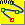Divide curve span ( by number or by distance)

Finds dividing points of a curve by distance or number.

Syntax:

PTObj.DivideCurveSpan( strObject, bMode, intNumber, doubleDis, bRound, bRoundMethod, bAdd )

Parameters:
 strObject String. Curve object to be divided bMode Boolean. 0 = divide by number, 1 = divide by distance intNumber Integer. Number of divide points doubleDis Double. Distance between dividing points bRound Boolean. Rounding to fit curve length bRoundMethod Boolean. true = round down. false = round up bAdd Boolean. Add dividing points to context
Returns:
 Array Array of divide points Null If not successful, or on errorDivide curve by distance on curve Finds dividing points of a curve by distance on curve.

Syntax:

PTObj.DivideCurveByDistance( strObject, doubleDis, bRound, bRoundMethod, bAdd )

Parameters:
 strObject String. Curve object to be divided doubleDis Double. Distance between dividing points bRound Bool. Rounding to fit curve length bRoundMethod Bool. True = round down. False = round up bAdd Bool. Add dividing points to context
Returns:
 Array Array of divide points Null If not successful, or on error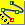Divide curve by exact or direct distance Finds dividing points of a curve by direct distance.

Syntax:

Parameters:
 strObject String. Curve object to be divided doubleDis Double. Direct distance between dividing points bAddEnd Bool. Add end point on curve bAdd Bool. Add dividing points to context
Returns:
 Array Array of divide points Null If not successful, or on error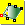Generate surface divide points based on surface UV directions Generates surface grid of points by number or distance following surface U & V directions. In case of dividing by distance, the defined distance is applied only on the first iso-curve in each direction. It varies if the surface is doubly curved.

Syntax:

PTObj.DivideSurfaceSpan( strSrfObject, bUMode,bVMode, intUNum, intVNum, doubleUDis, doubleVDis, bURound, bVRound, bURoundMethod, bVRoundMethod, bAdd )

Parameters:
 strSrfObject String. Surface object to generate point grid for bUMode Bool. Divide mode in U direction: False=By-Number, True=By-Distance bVMode Bool. Divide mode in V direction: False=By-Number, True=By-Distance intUNum Integer. Number of points in first ( U ) direction intVNum Integer. Number of points in second ( V ) direction doubleUDis Double. Distance between points in first ( U ) direction doubleVDis Double. Distance between points in second ( V ) direction bURound Bool. Option to round result in U direction bVRound Bool. Option to round result in V direction bURoundMethod Bool. True = round down. False = round up bVRoundMethod Bool. True = round down. False = round up bAdd Bool. Add points to context
Returns:
 Array of Arrays Array of point-objects arrays (2 dimensional array of points) Null If not successful, or on error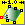Generate surface divide points based on direct distance Generates surface grid of points by direct distance between points. This method is a bit fragile. Each new point is based on previously calculated points. If a point fails to generate, subsequent points of that row fail as well. Setting extend option to true helps to create more predictable results in most cases (unless when extending the surface creates self intersecting or weird results).

Syntax:

PTObj.DivideSurfaceByDirectDistance( strSrfObject, doubleUDis, doubleVDis, bExtend, bAdd )

Parameters:
 strSrfObject String. Surface object to generate point grid for doubleUDis Double. Distance between points in first ( U ) direction doubleVDis Double. Distance between points in second ( V ) direction bExtend Bool. True = extend surface to possibly get better coverage bAdd Bool. Add points to context
Returns:
 Array Of Arrays Array of point-objects array (2 dimensional array of points) Null If not successful, or on errorSub paneling Paneling surface using seed polylines. Polylines are sub divided and pulled back to surfaces to generate panels.

Syntax:

PTObj.SubPaneling( strSrfObject, arrCrvObject, intMethod, intDegree, bPull )

Parameters:
 strSrfObject String. Surface object to be sub-paneled arrCrvObject Array of Strings. Polyline objects to sub-panel surface with intMethod Integer. 0=all, 1=subs only, 2=mains only intDegree Integer. Levels of sub paneling bPull Bool. Pull panels to surface or keep straight
Returns:
 Array Array of string of panel curves objects Null If not successful, or on error.Random paneling – Generate points randomly Triangular paneling using random set of points on surface.

Syntax:

PTObj.RandomPaneling( strSrfObject, intNumOfPoints, bPull )

Parameters:
 strSrfObject String. Surface object to be paneled randomly intNumOfPoints Integer. Number of points generated randomly on surface bPull Bool. Pull panels to surface or keep straight
Returns:
 Array Array of string of panel curves objects Null If not successful, or on error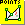Random paneling – Select points Triangular paneling using random set of points on surface.

Syntax:

PTObj.RandomPanelingWithPoints( strSrfObject, arrPointObjects, bPull )

Parameters:
 strSrfObject String. Surface object to be paneled randomly arrPoints Array. Points to be paneled bPull Bool. Pull panels to surface or keep straight
Returns:
 Array Array of string of panel curves objects Null If not successful, or on error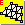Paneling edges of a grid Creates edge panels from an ordered paneling grid of points.

Syntax:

PTObj.PanelEdgesWithOrderedPoints( arrPoints, intPattern, [optional]strBrepObject, [optional] bPull )

Parameters:
 arrPoints Array. Points to be paneled intPattern Integer between 0 and 7. ( 0=Box, 1=BoxX, 2=Triangular, 3=Dense, 4=Diamond, 5=AngleBox, 6=Wave, 7=Brick ) strBrepObject [Optional] String. Base object (surface or polysurface) bPull [Optional] Bool. Pull panels to surface or keep straight
Returns:
 Array Array of string of curves objects (panels edges) Null If not successful, or on error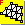Paneling faces of a grid Creates face panels (outline border) from an ordered paneling grid of points.

Syntax:

PTObj.PanelFacesWithOrderedPoints( arrPoints, intPattern, [optional]strBrepObject, [optional] bPull )

Parameters:
 arrPoints Array Points to be paneled intPattern Integer between 0 and 7. ( 0=Box, 1=BoxX, 2=Triangular, 3=Dense, 4=Diamond, 5=AngleBox, 6=Wave, 7=Brick ) strBrepObject [Optional] String. Base object (surface or polysurface) bPull [Optional] Bool. Pull panels to surface or keep straight
Returns:
 Array Array of string of curves objects (panels faces) Null If not successful, or on error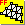Paneling flat faces of a grid Creates flat face panels (trimmed planar surfaces) from an ordered paneling grid of points.

Syntax:

PTObj.PanelFlatFacesWithOrderedPoints( arrPoints, intPattern, [optional]strBrepObject, [optional] intMethod )

Parameters:
 arrPoints Array. Points to be paneled intPattern Integer between 0 and 7. ( 0=Box, 1=BoxX, 2=Triangular, 3=Dense, 4=Diamond, 5=AngleBox, 6=Wave, 7=Brick ) strBrepObject [Optional] String. Base object (surface or polysurface) intMetod [Optional] Integer. 0=fit panel through corners, 1=align center to base surface
Returns:
 Array Array of string of surface objects Null If not successful, or on error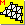Mesh of a grid Creates a mesh from an ordered paneling grid of points.

Syntax:

PTObj.PanelMeshWithOrderedPoints( arrPoints, intPattern, [optional]strBrepObject )

Parameters:
 arrPoints Array. Points to be paneled intPattern Integer between 0 and 7. ( 0=Box, 1=BoxX, 2=Triangular, 3=Dense, 4=Diamond, 5=AngleBox, 6=Wave, 7=Brick ) strBrepObject [Optional] String. Base object (surface or polysurface)
Returns:
 String Mesh object Null If not successful, or on error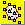Custom paneling of a grid Creates a custom pattern from pattern curves and points and ordered paneling grid of points. Pattern can be either curves, points, or both.

Syntax:

PTObj.PanelCustomWithOrderedPoints( arrPoints, arrPattern, intUSpacing, intVSpacing, [optional] strBrepObject, [optional] bPull )

Parameters:
 arrPoints Array. Points to be paneled arrPattern Array. Pattern curves and points intUSpacing Integer. Spacing in first or U direction intVSpacing Integer. Spacing in second or V direction strBrepObject [Optional] String. Base object (surface or polysurface) bPull [Optional] Bool. Pull panels to surface or keep straight
Returns:
 Array Array of string of curve objects Null If not successful, or on error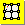Panel quads with ordered points Create quads point grid within deviation.

Syntax:

Parameters:
 arrPoints Array. Points to be paneled doubleDeviation Double. Maximum deviation from input grid
Returns:
 Array Array of point-objects Null If not successful, or on error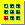Grid by array Create ordered grid of points by array.

Syntax:

PTObj.GridByArray( ptBase, intUNum, doubleUSpacing, vectorUdir, intVNum, doubleVSpacing, vectorVDir, bGroup, strName )

Parameters:
 ptBase Array. Base point of the array intUNum Integer. Number of points in first ( U ) direction doubleUSpacing Double. Distance between points in first ( U ) direction vectorVDir Array. First direction intVNum Integer. Number of points in second ( V ) direction doubleVSpacing Double. Distance between points in second ( V ) direction vectorVDir Array. Second direction bGroup Bool. Option to group result strName String. Grid name
Returns:
 Array Array of point-objects Null If not successful, or on errorGrid by array polar Create ordered grid of points by array polar.

Syntax:

PTObj.GridByArrayPolar( ptRotation, vectorRAxis, ptBase, vectorUDir, intUNum, doubleUSpacing, intVNum, doubleVAngle, bGroup, strName )

Parameters:
 ptRotation Array. Center of rotation vectorRAxis Array. Rotation axis ptBase Array. Base point of the array vectorVDir Array. Direction of points intUNum Integer. Number of points in first direction doubleUSpacing Double. Distance between points in first ( U ) direction intVNum Integer. Number of points in second ( polar ) direction doubleVAngle Double. Angle between points in second ( polar ) direction bGroup Bool. Option to group result strName String. Grid name
Returns:
 Array Array of point-objects Null If not successful, or on error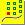Grid by one direction curves Create ordered grid of points using one directional curve.

Syntax:

PTObj.GridByOneDirectionCurve( strCrvObject, intUMethod, intUNum, doubleUDis, bURound, bURoundmethod, bUAddend, intVMethod, intVNum, doubleVDis, vectorVDir, bGroup, strName )

Parameters:
 strCrvObject String. Direction curve intUMethod Integer. Divide method of direction curve (0=ByNumber, 1=ByDistance, 2=ByDirectDistance) intUNum Integer. Number of divide points doubleUDis Double. Distance between divide points bURound Boolean. Rounding to fit curve length bURoundMethod Boolean. true = round down. false = round up bUAddEnd Boolean. Add end point (When divide by direct distance) intVMethod Integer. Extrude method in second ( V ) direction (0=Parallel, 1=Polar) intVNum Integer. Number of points in second ( V ) direction doubleVDis Double. Distance/Angle between points in second ( V ) direction vectorVDir Array. Second direction bGroup Bool. Option to group result strName String. Grid name
Returns:
 Array Array of point-objects Null If not successful, or on error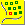Grid by two direction curves Create ordered grid of points using two directional curves.

Syntax:

PTObj.GridByTwoDirectionCurves( arrstrCrvs, intUMethod, intUNum, doubleUDis, bURound, bURoundmethod, bUAddend, intVMethod, intVNum, doubleVDis, bVRound, bVRoundmethod, bVAddend, bGroup, strName )

Parameters:
 arrstrCrvs Array of two Strings. Direction curves intUMethod Integer. Divide method of direction curve (0=ByNumber, 1=ByDistance, 2=ByDirectDistance) intUNum Integer. Number of divide points doubleUDis Double. Distance between divide points bURound Boolean. Rounding to fit curve length bURoundMethod Boolean. true = round down. false = round up bUAddEnd Boolean. Add end point (When divide by direct distance) intVMethod Integer. Divide method of second curve (0=ByNumber, 1=ByDistance, 2=ByDirectDistance) intVNum Integer. Number of divide points doubleVDis Double. Distance between divide points bVRound Boolean. Rounding to fit curve length bVRoundMethod Boolean. true = round down. false = round up bVAddEnd Boolean. Add end point (When divide by direct distance) bGroup Bool. Option to group result strName String. Grid name
Returns:
 Array Array of point-objects Null If not successful, or on error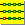Grid by U curves Create ordered grid of points using array of curves.

Syntax:

PTObj.GridByUCurves( arrstrCrvs, intMethod, intNum, doubleDis, bRound, bRoundmethod, bAddend, bGroup, strName )

Parameters:
 arrstrCrvs Array of Strings. Curves in order intMethod Integer. Divide method of curves (0=ByNumber, 1=ByDistance, 2=ByDirectDistance) intNum Integer. Number of divide points doubleDis Double. Distance between divide points bRound Boolean. Rounding to fit curve length bRoundMethod Boolean. true = round down. false = round up bAddEnd Boolean. Add end point (When divide by direct distance) bGroup Bool. Option to group result strName String. Grid name
Returns:
 Array Array of point-objects Null If not successful, or on error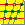Grid by UV curves Create ordered grid of points using array of curves in two directions. Grid is made out of curves intersections.

Syntax:

PTObj.GridByUVCurves( arrstrCrvs0, arrstrCrvs1, bGroup, strName )

Parameters:
 arrstrCrvs Array of Strings. Curves of first direction, in order arrstrCrvs Array of Strings. Curves of second direction, in order bGroup Bool. Option to group result strName String. Grid name
Returns:
 Array Array of point-objects Null If not successful, or on error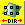Grid by object direction Create ordered grid of points using base object and direction curve.

Syntax:

PTObj.GridByObjectDirection(strBrepObject, strCrvObject, vectorProjDir, vectorExtrudeDir, intCutNum, doubleCutDis, intMethod, intNum, doubleDis, bRound, bRoundmethod, bAddend, bGroup, strName )

Parameters:
 strBrepObject String. Object to create grid for strCrvObject String. Direction curve vectorProjDir Array(x,y,z). Projection direction vectorExtrudeDir Array(x,y,z). Extrusion direction intCutNum Integer. Number of cuts doubleCutDis Double. Distance between cuts intMethod Integer. Divide method of curves (0=ByNumber, 1=ByDistance, 2=ByDirectDistance) intNum Integer. Number of divide points doubleDis Double. Distance between divide points bRound Boolean. Rounding to fit curve length bRoundMethod Boolean. true = round down. false = round up bAddEnd Boolean. Add end point (When divide by direct distance) bGroup Bool. Option to group result strName String. Grid name
Returns:
 Array Array of point-objects Null If not successful, or on error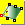Grid by surface UV Create ordered grid of points following input surface UV direction.

Syntax:

PTObj.GridBySurfaceUV( strSrfObject, bUMode, bVMode, intUNum, intVNum, doubleUDis, doubleVDis, bURound, bVRound, bURoundMethod, bVRoundMethod, bGroup, strName )

Parameters:
 strSrfObject String. Surface object to generate point grid for bUMode Bool. Divide mode in U direction: False=By-Number, True=By-Distance bVMode Bool. Divide mode in V direction: False=By-Number, True=By-Distance intUNum Integer. Number of points in first ( U ) direction intVNum Integer. Number of points in second ( V ) direction doubleUDis Double. Distance between points in first ( U ) direction doubleVDis Double. Distance between points in second ( V ) direction bURound Bool. Option to round result in U direction bVRound Bool. Option to round result in V direction bURoundMethod Bool. True = round down. False = round up bVRoundMethod Bool. True = round down. False = round up bGroup Bool. Option to group result strName String. Grid name
Returns:
 Array Array of point-objects Null If not successful, or on error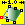Grid by direct distance on surface Create ordered grid of points using input surface and distance between points.

Syntax:

PTObj.GridByDirectDistanceOnSurface( strSrfObject, doubleUDis, doubleVDis, bExtend, , bGroup, strName )

Parameters:
 strSrfObject String. Surface object to generate point grid for doubleUDis Double. Distance between points in first ( U ) direction doubleVDis Double. Distance between points in second ( V ) direction bExtend Bool. True = extend surface to possibly get better coverage bGroup Bool. Option to group result strName String. Grid name
Returns:
 Array Array of point-objects Null If not successful, or on error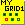Insert a point in a grid This is useful for building custom grid of points.

Syntax:

PTObj.InsertPointInGrid( ptPoint, intRow, intCol, [optional] strName )

Parameters:
 ptPoint Array. Point to be added to a grid intRow Integer. Row index of the point intCol Integer. Column index of the point strName [optional]String. Grid name
Returns:
 String Point object Null If not successful, or on error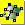Surface by edit point grid Create a NURBs surface from a point grid.

Syntax:

PTObj.SurfaceByGrid( arrGrid grid )

Parameters:
 arrGrid Array. Array of paneling point objects (ordered points)
Returns:
 String Surface object Null If not successful, or on error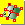Surface by control point grid Create a NURBs surface from a grid of control points.

Syntax:

PTObj.SurfaceByControlGrid( arrGrid )

Parameters:
 arrGrid Array. Array of paneling point objects (ordered points)
Returns:
 String Surface object Null If not successful, or on error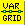Divide Curve with Variable Distances Use a list of parameters to divide a curve.

Syntax:

Parameters:
 strCrvObj Curve object arrParamList Array. Array of dividing parameters on curve bAdd Boolean. Add dividing points to context
Returns:
 Array Array of divide points Null If not successful, or on errorDivide Surface with Variable Distances Use a list of parameters in U and V directions to divide a surface.

Syntax: